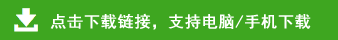# 一年级数学下册期末测试卷（附参考答案）

1.17-9=              2.5+43=              3.98-7=               4.79-60=
5.55+7=              6.47+30=             7.63-6=               8.78-30=
9.65-7=             10.82-5=              11.36+7=              12.47+50=
13.17+6+9=          14.43-3-20=           15.60+38-9=           16.68+7-9=

1.和80相邻的两个整十数是(    )和(    )
49 53
2.
67 64
3.最大的—位数是(    )，最小的两位数是(    )，它们的和是 (    )，差是(    )。
4.50添上(    )个十是80。
5.9个十9个一合起来是(    )，和它相邻的数是(    )和(    )。
6.40 比64少(    )，66 比6多(    )。
7.32角=(    )元(    )角     5元=(    )角
3.60元=(    )元(    )角    100分=(    )角
8.被减数和减数都是30，差是(    )，再加上15，和是(    )。
9.我在班上的座位是第(    )排第(    )个。我前面有(    )名同学。后面有(    )名同学。
10.我用1张5元钱买一根1元5角钱的冰棒，应找回(    )钱。